# Comparing RF power measurements using power sensors and spectrum analysers

How your approach can influence outcomes in RF and microwave power measurement

By Giovanni D’Amore, Marketing Brand Manager, Keysight Technologies

The ability to make consistent measurements of RF or microwave power levels is vital to developing efficient, effective radio systems and networks. Making these measurements demands an informed choice of equipment and approach, to ensure that the advantages and limitations of each do not undermine the validity and utility of the measurements.

This article explores the impact of different approaches, one using a power sensor and the other a spectrum analyser, to make average power measurements of continuous wave (CW), multi-tone, 32 QAM (quadrature amplitude modulation), and pulsed signals.

The test signals came from a Keysight MXG vector signal generator producing a 6 GHz signal with an output power of -20 dBm. The power sensor measurements used a Keysight U2000A USB power sensor connected to an N9938A FieldFox spectrum analyser with Option 302. The spectrum-analyser based approach used the same Fieldfox system, configured with a built-in channel power meter (CPM, Option 310), connected to the signal generator over coaxial cable.

Measuring a CW signal

CW signals do not carry any information and so the average power and peak power are the same and the signal has no bandwidth. This means that measurement equipment can use a narrow filter to improve its sensitivity (noise floor).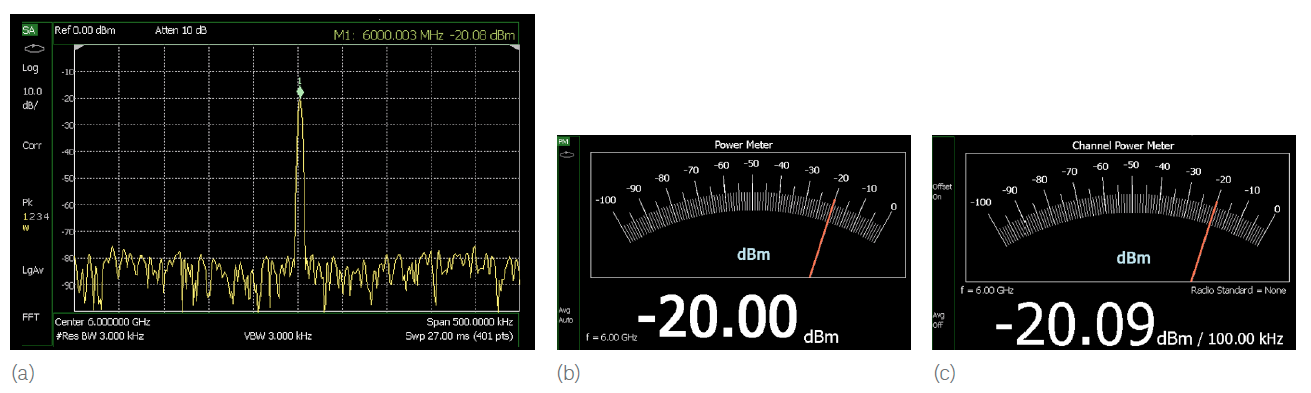Figure 1: Measuring a CW signal using (a) spectrum analyser and marker, (b) power sensor and (c) spectrum analyser with CPM (Source: Keysight Technologies)

Figure 1a shows the swept frequency response of a typical spectrum analyser, around a single tone across a measurement span of 500 kHz. The signal’s peak power (which is also its average power) is given as -20.08 dBm. Figure 1b shows the average power measured by the USB power sensor as -20.00 dBm. Figure 7c shows the average power measured using the CPM option on the FieldFox as -20.09 dBm.

The three measurements are within 0.1 dB of each other, with the power sensor having the highest accuracy, or lowest measurement uncertainty.

The FieldFox’s built-in CPM measurement system has to have a span set for it. This is equivalent to the channel bandwidth of the signal under test. In the Figure 1c measurement, the span was set to 100 kHz. Since a CW signal has no bandwidth, the span can be set to any value, although a narrow span increases measurement time while a wider span increases noise and uncertainty.

Measuring a multi-tone signal

In this example, the power from the signal generator is distributed among five tones, spaced at 500 kHz intervals.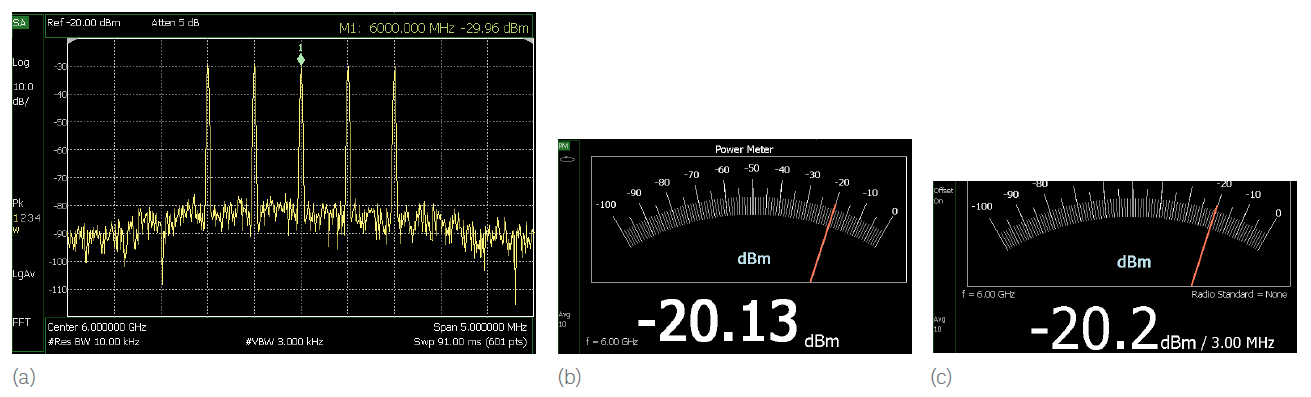Figure 2: Measuring a multi-tone signal using the (a) spectrum analyser, (b) power sensor and (c) spectrum analyser with CPM (Source: Keysight Technologies)

If the total power for the tones is -20 dBm (10 μW) then each has a power of ‑26.98 dBm (2μW). An average power sensor will measure the average power of all five tones, in this case, -20.13 dBm. The CPM gives a measurement of ‑20.2 dBm, so long as the CPM’s measurement span has been set to include all five tones (a span of at least 2.5 MHz, in this case). If the CPM-based measurement was repeated with a span of just 200 kHz, only the central tone at 6 GHz would be included, giving a power measurement of -27.1 dBm — close to the theoretical value of -26.98 dBm for a single tone.

Making measurements of single tones or specific measurement spans is only possible with a spectrum analyser, since power sensors are not frequency selective.

The peak power of this signal is not the same as its average power, because of the phase relationship between the tones. Peak power measurements usually need a sensor with peak power capability and a filter wide enough to process all the tones at once.

Measuring a digitally modulated signal

The digitally modulated signal shown here uses 32 QAM with a symbol rate of 5 Msymbol/s. The symbols are filtered, so the signal bandwidth is approximately 8 MHz (see Figure 3a). The power sensor reports an average power of -20.00 dBm (Figure 3b) and the CPM reports -20.0 7dBm (Figure 3c).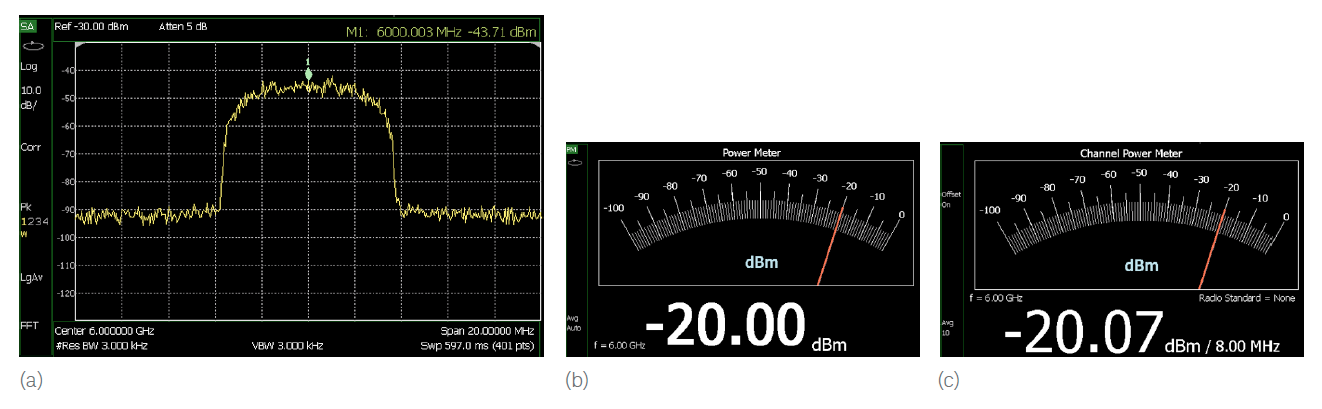Figure 3: Measuring a 32 QAM signal using the (a) spectrum analyser, (b) power sensor, and (c) tuned receiver with CPM (Source: Keysight Technologies)

A power sensor will report the correct average power of a signal with unknown bandwidth so long as the signal’s power level and frequency range are within the sensor’s capabilities. On the other hand, the correct measurement span must be set on the CPM in the FieldFox to make accurate measurements. To do this, you can use a standard spectrum analyser to discover the bandwidth of the unknown signal, and then switch back to the CPM once it is configured with the span setting to match.

Measuring pulsed waveforms

In pulsed waveforms, the ratio of the pulsewidth (how long the pulse is on) to the time between the pulses (its period) is known as the duty factor (DF). The peak power of such waveforms is the average power divided by the DF, given a repeating pulse-train.

Figure 4 shows an example of the measured spectrum and average power of a pulsed waveform with a 20 μs pulse width and a 20% DF.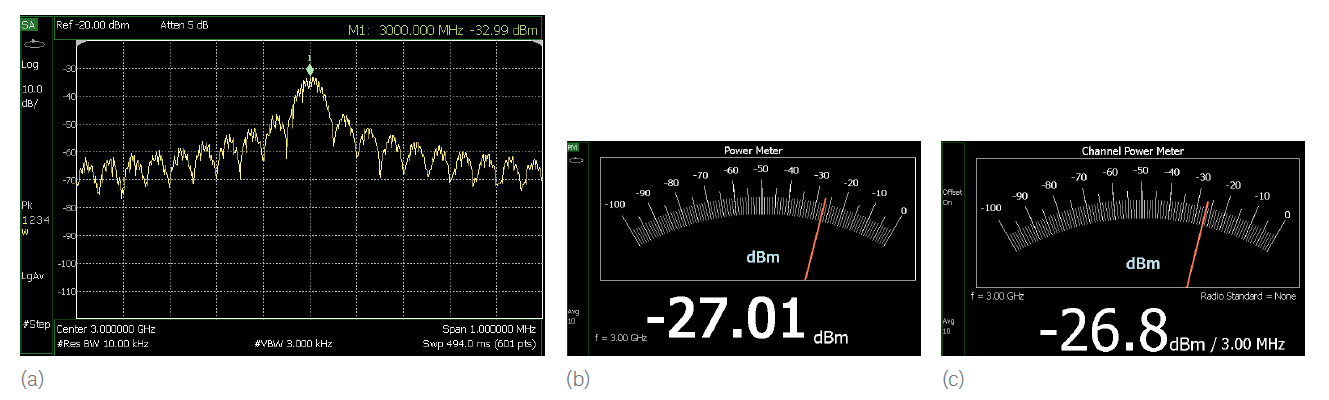Figure 4: Measuring a pulsed waveform with 20% duty factor using the (a) spectrum analyser, (b) power sensor and (c) spectrum analyser with CPM (Source: Keysight Technologies)

The spectrum analyser display in Figure 4a shows a typical “sin(x)/x” response as a function of frequency. The sidebands in the frequency domain continue outside the displayed 1 MHz frequency span. The average power measurement using the power sensor, as shown in Figure 4b, reports a power of -27.01 dBm. The measurement using the CPM reports an average power of –26.8 dBm (Figure 4c).

For the CPM measurement, the measurement span was set to 3 MHz to capture most of the waveform’s sideband energy. Increasing the span to more than 3 MHz did not change the measured power. The peak power for this waveform with a 20% DF can be calculated by increasing the average level by 6.99 dB (10log(1/DF)). Using a measured average power of -27 dBm, the peak power is calculated to be -20 dBm — which is what the signal generator was set to produce.

Detection and filtering in peak power measurements

The block diagram for a power sensor and meter designed to measure peak power is similar to that for an average power sensor (see Figure 5). The peak power sensor/meter will have a wider bandwidth and higher sample rates in its ADC, to capture the rapid transitions found in pulsed and complex modulated waveforms.

In practice, a typical peak power sensor has two measurement paths, optimised for measuring either the average power or the peak power.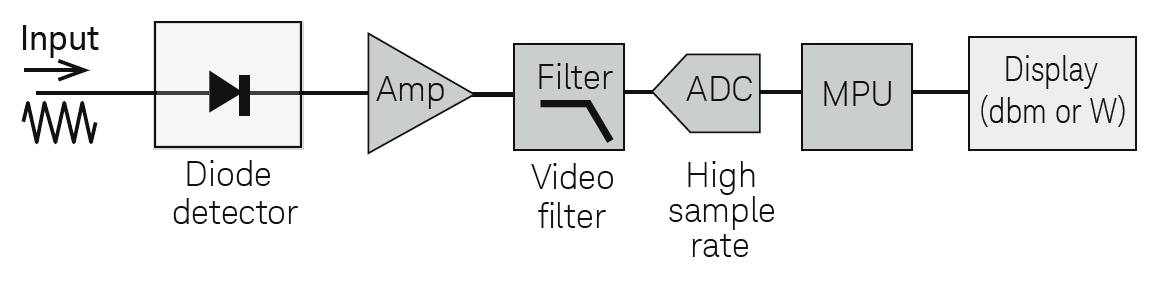Figure 5: Simplified block diagram of a peak power sensor/meter (Source: Keysight Technologies)

In the peak power measurement path, the sensor has a two-layer detection scheme.

The first detection occurs at the front end where the diode rectifies the incoming waveform. As the diode detector is usually a wideband device, the rapidly changing envelope of the pulsed waveform is preserved in the rectified waveform. After detection, the signal is amplified and filtered. The filter’s bandwidth is likely to be selectable, at up to 30 MHz for the Keysight P-Series and X-Series sensors, and has to be greater than the signal under measurement.

The second detection layer occurs after filtering, where the ADC samples the signal at up to 1 Gsample/s (in peak power meters such as the Keysight 8990). This sampling rate is necessary to capture the shape of the waveform, from which the peak power, pulsewidth, period, rise time, and fall time characteristics can be measured or derived.

Peak power sensors can also interface with power meters, spectrum analysers, and laptops for field measurements. The next section shows how a peak power sensor connected to a portable spectrum analyser can be used to measure the time domain profile of a pulsed waveform.

Making measurements with a peak power sensor

Figure 6 shows an example of the measured power envelope of a pulsed waveform as a function of time.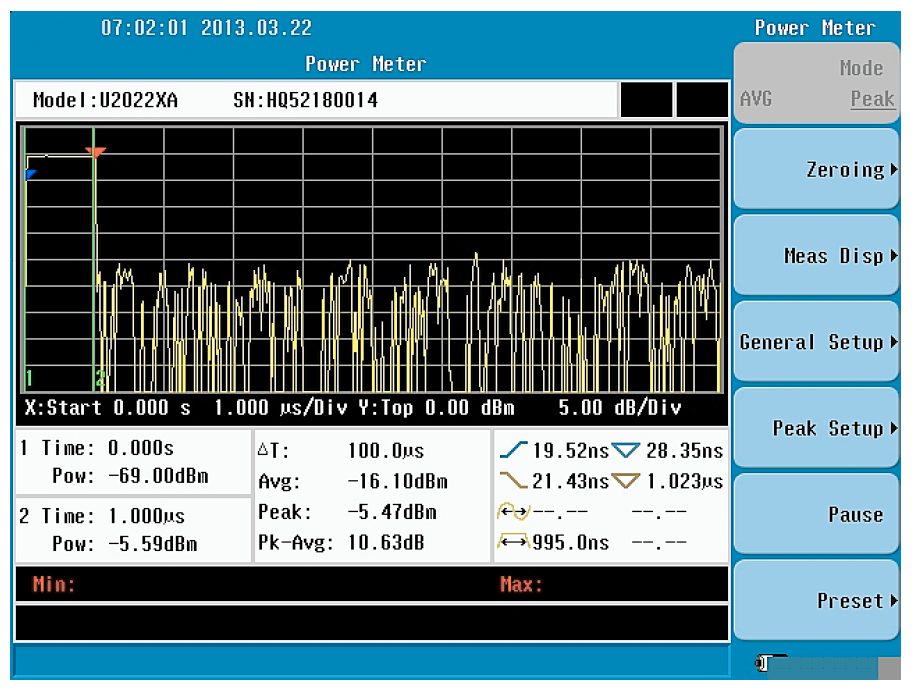Figure 6: Measuring a pulsed waveform using a peak power sensor connected to a spectrum analyser (Source: Keysight Technologies)

The input waveform is a pulse-modulated 40 GHz signal with a 1 μs pulse width and 10% DF. It was captured using a Keysight N9344C handheld spectrum analyser connected to a U2020 X-Series USB peak and average power sensor. In this set-up, only the power sensor needs to be rated to 40 GHz, as the spectrum analyser is only used as a display and control interface.

Using this type of peak power sensor, an analyser or peak power meter can display peak power, average power, and waveform characteristics such as rise and fall times. Markers on the instrument display can show specific timing and power levels in the captured waveform. The Keysight peak power meters have bandwidths of up to 30 MHz, making it possible to measure waveforms’ rise and fall times down to 13 ns.

Conclusions

Modern equipment, be it a standalone power sensor or power meter, or a spectrum analyser with CPM, can make sophisticated measurements on complex signals. However, there are nuances to the way each works that need to be understood if the user is going to be confident in the measurements made in each context.